Radeva, G., Valchev, I., Petrin, S., Valcheva, E., and Tsekova, P. (2012). "Kinetic study of the enzyme conversion of steam exploded Paulownia Tomentosa to glucose," BioRes. 7(1), 412-421.

#### Abstract

Paulownia Tomentosa was pretreated by steam explosion. The cellulase complex NS 50013 and the β-glucosidase NS 50010 of Novozymes AS were used for the enzymatic conversion of cellulose to glucose. The kinetics of enzyme conversion was studied using the exponential kinetic equation valid for processes taking place at uniformly inhomogeneous surfaces. The kinetic coefficient of inhomogeneity accounts for the energy and entropy inhomogeneity of the system and depends on the temperature. It was established that both the activation energy and the pre-exponential factor increase simultaneously with increasing of conversion degree. A compensation effect between pre-exponential factor and activation energy was observed. The energetic hindrances established cannot be completely compensated by the positive effect of the pre-exponential factor increase. Hence, the activation energy has a determining influence on how quickly the rate of hydrolysis decreases.

KINETIC STUDY OF THE ENZYME CONVERSION OF STEAM EXPLODED PAULOWNIA TOMENTOSA TO GLUCOSE

Greta Radeva,* Ivo Valchev, Stoiko Petrin, Eva Valcheva, and Petya Tsekova

Paulownia Tomentosa was pretreated by steam explosion. The cellulase complex NS 50013 and the β-glucosidase NS 50010 of Novozymes AS were used for the enzymatic conversion of cellulose to glucose. The kinetics of enzyme conversion was studied using the exponential kinetic equation valid for processes taking place at uniformly inhomogeneous surfaces. The kinetic coefficient of inhomogeneity accounts for the energy and entropy inhomogeneity of the system and depends on the temperature. It was established that both the activation energy and the pre-exponential factor increase simultaneously with increasing of conversion degree. A compensation effect between pre-exponential factor and activation energy was observed. The energetic hindrances established cannot be completely compensated by the positive effect of the pre-exponential factor increase. Hence, the activation energy has a determining influence on how quickly the rate of hydrolysis decreases.

Keywords: Paulownia; Cellulase; Kinetics; Exponential equation; Compensation effect

Contact information Contact information: University of Chemical Technology and Metallurgy, 8, St. Kliment Ohridski blvd., 1756, Sofia, Bulgaria; *Corresponding author: grradeva@abv.bg

INTRODUCTION

Conversion technologies for producing ethanol from cellulose biomass resources such as forest materials, agricultural residues, and urban wastes are under development and have not yet been demonstrated commercially (Derimbas 2005). Biomass from plan-tations of fast growing tree species is suitable for processing to bioethanol. Paulownia is a deciduous tree capable of achieving very high growth rates under favourable conditions.

In order to produce bioethanol from cellulosic biomass, a pretreatment process is used to reduce the particle size, open up the structure of the cellulose component, and allow enzymatic reactions to occur, breaking down the cellulose to glucose. Steam explosion (autohydrolysis) is one of the most cost effective and widely used pretreatment methods for cellulose biomass (Alfani et al. 2000). According to this method size-reduced biomass is rapidly heated by high-pressure steam for a short period of time, and then the pressure is suddenly reduced, which makes the materials undergo an explosive structural breakdown (Jurado et al. 2009).

Hydrolysis using appropriate enzymes represents the most effective method to liberate simple sugars from cellulosic materials. Cellulose hydrolysis is catalyzed by a class of enzymes known as cellulases. Three major groups of enzymes namely, endo-gluconase, exo-gluconase, and -glucosidase are involved in hydrolysis of cellulose. The enzymatic hydrolysis can be influenced by substrate and end product concentrations, enzyme activity, and reaction conditions. – glucosidase plays a significant role in the hydrolysis process, since cellobiose is an end-product inhibitor of many cellulases (Talebnia et al. 2010; Galbe and Zacchi 2002). The products of the hydrolysis are usually reducing sugars, including glucose.

The susceptibility of cellulosic substrates to cellulases depends on the structural features of the substrate, including cellulose crystallinity, degree of cellulose polymerize-tion, surface area, and content of lignin. The content of non-crystalline domains has the highest effect on the average conversion degree of celluloses at the enzymatic hydrolysis (Ioelovich et al. 2011).

Enzymatic hydrolysis is a hurdle because of the heterogeneous nature of the cellulose substrate and the fact that degradation of the cellulose chain progresses in only one direction for each cellobiohydrolase, effectively reducing the reaction to a one-dimensional process. Cellulose is known to consist of relatively easily accessible amorphous regions with few lateral interactions between the cellulose chains as well as of crystalline domains that are much harder to hydrolyze. In addition, the hydrolysis product of the cellulase reaction, cellobiose (glucosyl -1-4 glucose), is well known to severely inhibit cellulase. Therefore, -glucosidase is added to the cleave cellobiose to glucose (Bommarius et al. 2008).

Cellulose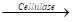cellobiose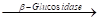glucose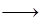EtOH (1)

Optimization of lignocellulosic bioconversion by cellulase enzymes requires good knowledge of the reaction kinetics. The complexity of the enzymatic hydrolysis of lignocellulosic biomass comes from the fact that they are heterogeneous, insoluble substrates, and thus, their enzymatic hydrolysis is always limited (Carrillo 2005). The enzyme kinetics has been usually studied by the Henri-Michaelis-Menten equation (Henri 1902). However, it has been shown that the Henri-Michaelis-Menten equation is not suitable for the analysis of enzymatic reactions of heterogeneous systems (Chrastil and Wilson 1982; Chrastil 1988a; Baley 1989).

The heterogeneous nature of the system Paulownia – cellulase makes it possible to apply the models of heterogeneous catalytic reactions for description of the kinetics of enzymatic conversion of cellulose to glucose, which is the purpose of this study.

EXPERIMENTAL

The investigations were performed on wood species of Paulownia tomentosa. The chemical composition of Paulownia was as follows: 39.2% of cellulose (Kurshner and Hoffer 1933), 23.8% pentosans (TAPPI standard T19m-54); 20.2% lignin (TAPPI standard T222 om-88); and 4.6% of ash.

The steam explosion method was used as a pretreatment of paulownia. The steam explosion was performed in a 2 L stainless steel laboratory installation at the following conditions: a hydromodul ratio 1:10; an initial temperature of 100°C; a maximum temperature of 190°C; pressure of 12.8 bar; and heating time of 60 min, followed by additional 10 min at maximum temperature.

The residue was washed with distilled water, and the obtained hydrolysate was filtered. The solid residue produced in the course of steam explosion pretreatment was subjected to enzymatic hydrolysis as a second stage of treatment.

The cellulase complex NS 50013 with activity 700 EGU/g (70 FPU/g) and β-glucosidase NS 50010 with activity 250 CBU/g of Novozymes AS were used for the enzymatic hydrolysis. The enzyme charge of NS 50013 was 5%, while that of NS 50010 was 0.5%, both referred to the mass. All amounts and experiment conditions were according to the Novozymes Application Sheet.

The cellulasic hydrolysis was carried out in polyethylene bags in a water bath previously heated to the desired temperature.

The kinetics of the cellulasic hydrolysis was examined at temperatures of 20°C, 30°C, 40°C, and 50°С, consistency of 10 %, рНinitial 5.0 to 6.0, рНfinal 4.2 to 4.6, and reaction time of 1 to 24 hours.

The glucose content was analyzed using a Dionex HPLC system according to NREL standard biomass analytical procedure (Sluiter et al. 2006). The amount of glucose formation was calculated in percent with respect to the mass.

RESULTS AND DISCUSSION

Paulownia tomentosa was preliminary treated by steam explosion. The resulting dissolved substances in the filtrate amounted to 32.6%, a sum comprised of the following components: glucose – 3.7%; xylose – 1.1%; galactose – 2.7%; arabinose – 0.6%; fufural – 0.06%, hydroxyl-methyl- furfural (HMF) – 0.05%, and reducing sugars – 13.8%.

The preliminary treated Paulownia is followed by enzyme hydrolysis using the enzyme cellulase complex NS 50013 and β – glucosidase NS 50010. The amounts of glucose obtained G at different temperatures and reaction times are presented in Table 1.

Table 1. Dependence of Glucose Amount G, % on the Temperature and Time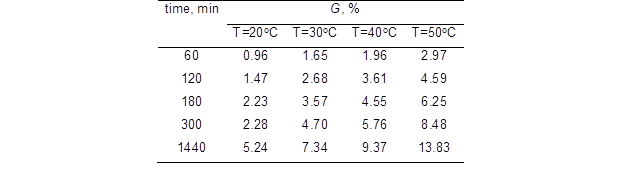The dimensionless quantity is used as a kinetic variable and has the meaning of a degree of hydrolysis, i.e. degree of conversion of cellulose to glucose. It is determined by the relative change of the glucose amount and is calculated in correspondence with Eq. (2),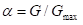(2)

where G (%) is the current amount of glucose obtained, while Gmax (%) is the maximal amount (equal to 22.32%) obtained after 48 hours of treatment at a temperature of 50°C. The data received was used to present the corresponding kinetic curves at different temperature values (see Fig. 1). The degree of conversion increased with increasing time and with increasing temperature.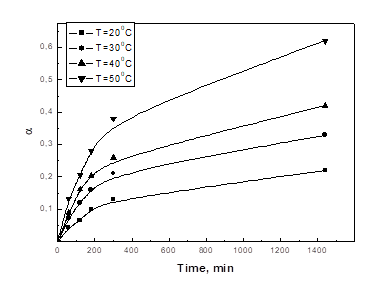Fig. 1. Kinetic curves of degree of enzyme hydrolysis increasing at different temperatures

The applicability of different kinetic equations valid for heterogeneous processes was verified (Valcheva et al. 2003). It was found that the enzyme process was best described by an exponential kinetic equation, which yielded a higher coefficient of determination (R2=0.998). The exponential kinetic equation is valid for processes taking place at uniformly inhomogeneous surfaces. According to the model of uniformly inhomogeneous surfaces the active centers on the surface are distributed linearly referring to their energy and entropy,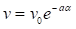(3)

where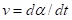is the current rate and v0 is the initial rate of enzymatic hydrolysis. The kinetic coefficient of inhomogeneity а accounts for the energy and the entropy inhomogeneity of the system (Veleva et al. 2001; Valcheva et al. 2003). In the general case it depends on the temperature according to the following equation: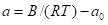(4)

The constant B describes the interval of energy inhomogeneity that takes into account the presence of active centers with different energy value. The constant a0 characterizes the entropy inhomogeneity of the surface, which is due to the changing number of active centers in the course of the process, as well as their accessibility and spatial orientation.

All kinetic curves were linearized in coordinates vs. ln t in correspondence with the approximate integral form of the exponential kinetic equation (Eq. 3):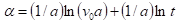(5)

The linear dependences obtained are presented in Fig. 2.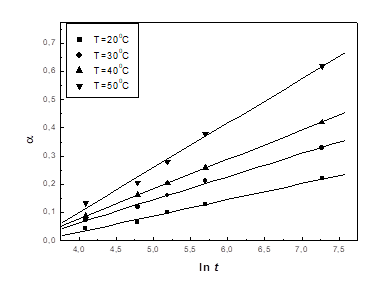Fig. 2. Linear dependences vs ln t

The kinetic coefficient of inhomogeneity a, which depends on the temperature and supposes energy and entropy inhomogeneity of the system, was determined. This dependence is presented on the Fig. 3.

The values of the constants in Eq. 3, which are respectively: for the interval of energy inhomogeneity B=251, kJ/mol, and for the interval of entropy inhomogeneity a0=88.3, were calculated.

Equations (5) and Eq. (3) were used to estimate the initial v0 and current v rates of the hydrolysis process, respectively. The values obtained are summarized in Table 2. It is seen that the current rate decreased in the course of the process (with increase).

Table 2. Dependence of the Hydrolysis Rate v, min-1 on at Different Temperature Values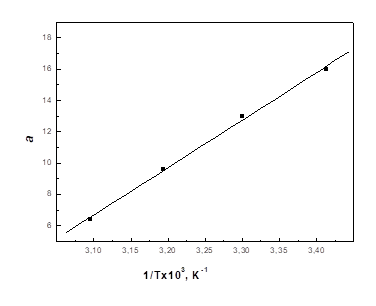Fig. 3. Temperature dependence of coefficient of inhomogeneity a

The temperature dependence of the hydrolysis rate was followed in correspond-dence with the Arrhenius equation, which can be presented in the following form (Eq. 6),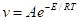(6)

where A is the pre-exponential factor and E is the activation energy. The linear dependences, in accordance with the logarithmic form of Eq. 6, are shown in Fig. 4.

The activation energy Е and the pre-exponential factor А were calculated at different constant values ofand they are presented in Table 3.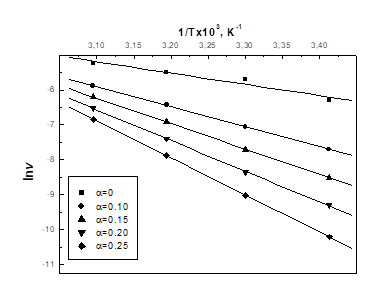Fig. 4. Temperature dependence of the initial and current rate of the process at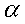=const.

Table 3. Values of Activation Energy E and Pre-exponential Factor lnA atconst.The values of the activation energy and the pre-exponential factor increased in the course of the process, as it is seen from Table 3. Their dependences on the degree of hydrolysis were linear, as required by the model of inhomogeneous surfaces (Radeva et. al 2009, 2010a,b) and are described by Eqs. (7) and (8),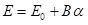(7)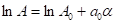(8)

where E0 and ln Aare calculated from the temperature dependence of the initial rate v0.

The increase of the activation energy E in the course of the enzymatic hydrolysis shows that the process is delayed due to the increase of the energy difficulties of the enzyme – cellulose system. The effect observed may be explained as being due to conformation changes in the enzymes cellulase and β – glucosidase and their respectively reduced activity when interacting in areas difficult to access within the cell walls. On the other hand, the pre-exponential factor A also increases in the course of the process. This is probably due to the fact that the enzyme hydrolysis of Paulownia is a complex two-stage process. The pre-exponential factor accounts not only for the newly formated active centers on the cellulose surface but also for the total amounts of cellobiose and oligosaccharides, which increase in the course of the process.

The process rates are affected both by the activation energy and the pre-exponen-tial factor. When these counteracting factors are changed simultaneously with increase, then a well-known effect is observed, the heterogeneous kinetics compensation effect, which relates lnA and E in one common linear dependence (Eq. 9), which is presented in Fig. 5,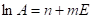(9)

where the coefficient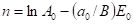is connected with the initial characteristics of the process and coefficient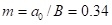, mol/kJ is the ratio between entropy and energy inhomogeneity of the system.

The energetic hindrances established cannot be completely compensated by the positive effect of the pre-exponential factor increase. Hence, the activation energy has a determining influence on decreasing of the rate as a function of time.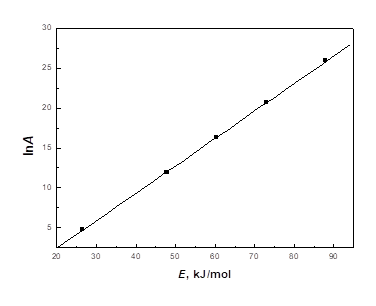Fig. 5. Compensation effect

CONCLUSIONS

The kinetics of enzyme conversion of cellulose to glucose was studied. The exponential kinetic equation valid for processes taking place at uniformly inhomogeneous surfaces was applied. The kinetic coefficient of inhomogeneity, which depends on temperature and takes into account the energy and entropy inhomogeneity, was determined.

It was established that the activation energy increases in the course of the process because of conformational changes in the enzyme and reduction of its activity when interacting in difficult-to-access areas of the cell walls. The pre-exponential factor A also increases in the course of the process because of increasing of the active centers on the cellulose surface and the amount of cellobiose and oligosaccharides.

A compensation effect between pre-exponential factor and activation energy was observed. The activation energy has a determining influence on the decreasing of the rate as a function of time.

ACKNOWLEDGMENTS

The authors thank the National Science Fund, Ministry of Education and Science, Bulgaria for the financial support of these investigations.

REFERENCES CITED

Alfani, F., Gallifouco, A., Saporosi, A., Spera, A., and Cantarella, M. (2000). “Comparison of SHF and SSF processes for bioconversion of steam-exploded wheat straw,” J. Ind. Microbiol. Biot. 121, 1133-1141.

Baley, D. (1989). “Enzyme kinetics of cellulose hydrolysis,” Biochem. J. 262, 1001-1002.

Bommarius, A., Katona, A., Cheben, S., Patel, A., Ragauskas, A., Knudson, K., and Pu, Y. (2008). “Cellulase kinetics as a function of cellulose pre-treatment,” Metab. Eng. 10, 370-381.

Carrillo, F., Lis, M. J., Colom, X., Lopez-Mesas, M., and Vallderas, J. (2005). “Effect of alkali pretreatment on cellulase hudrolysis of wheat straw: Kinetic study,” Process Biochem. 40, 3360-3364.

Chrastil, J. (1988). “Enzymatic product formation curves with the normal or diffusion limited reaction mechanism and in the presence of substrate receptors,” Int. J. Biochem. 20, 683-93.

Chrastil, J., and Wilson, J. T. (1982). “The effect of chemical and physiological factors on the kinetics for products formation as it relates to enzyme activity and concentration, reaction time and to substrate adsorption and affinity,” Int. J. Biochem. 14, 1-17.

Derimbas, A. (2005). “Bioethanol from cellulosic materials: A renewable motor fuel from biomass,” Energy Sources Part A 27, 327-337.

Galbe, M., and Zacchi, G. (2002). “A review of the production of ethanol from softwood,” Appl. Microbiol. Biot. 59, 618-628.

Henri, V. (1902). “Theorie generale de l’action de quelque diastases,” Comptes Rendus Hebdomadaires des Seances de L Academie des Science. 135, 916-919.

Ioelovich, M., and Morag, E. (2011) “Effect of cellulose structure on enzymatic hydrolysis,” BioResources (http://ncsu.edu/bioresources), 6(3), 2818-2835.

Jurado, M., Prieto, A., Martinez-Alcala, A., Martinez, A., and Martinez, M. (2009). “Laccase detoxification of steam explosion wheat straw for second generation bioethanol,” Bioresource Technol. 100, 638-684.

Kurschner, K., Hoffer, A. (1933) “Cellulose and cellulose derivate,” Fresenius J. Anal. Chem. 92, 145-154.

Radeva, G., Valcheva, E., and Veleva S. (2010b). “A correlation method in studying chemisorption processes on uniformly inhomogeneous surfaces,” Adsorpt. Sci. Technol. 28, 149-162.

Radeva, G., Bikov, P., and Valchev, I. (2009). “Kinetic model of cellulose treatment of pulp,” Italic 5, M. Orlandi and C. Crestini (Eds) Science &Technology of Biomasses, Proceeding book, Sept 1-4, Varena (Como), Italy, p. 129-132.

Radeva, G., Valchev, I., and Tsekova, P. (2010a). “Kinetics of enzyme hydrolysis of lignocellulosic raw materials,” XXI TECNICELPA Conference and Exhibition / VI Congress CIADICYP, Editor TECNICELPA, 12-15 October, Lisbon, Portugal, p. 75

Sluiter, A., Hames, B., Ruiz, R., Scarlata, C., Sluiter, J., and Templeton, D. (2006). “Determination of sugars, bioproducts and degradation products in lignin fraction process samples,” Laboratory Analytical Procedure, National Renewable Energy Laboratory (LAP), Issue Date 12/08/2006.

Talebnia, F., Karakashev, D., and Angelidaki, I. (2010). “Production of bioethanol from wheat straw: An overview on pre-treatment, hydrolysis and fermentation,” Bioresource Technol.101, 4744-4753.

Valcheva, E., Veleva, S., Radeva, G., and Valchev, I. (2003). “Enzyme action of the laccase-mediator system in the pulp delignification process,” React. Kinet. Catal. Lett. 78 , 183-191.

Veleva, S., Valcheva, E., Valchev, I., and Radeva G. (2001). “Application of an exponential kinetic equation to the interaction of optical brighteners with pulp,” React. Kinet. Catal. Lett.72, 355-364.

Article submitted: September 2, 2011; Peer review completed: September 24, 2011; Revised version received and accepted: November 15, 2011; Published: November 18, 2011.